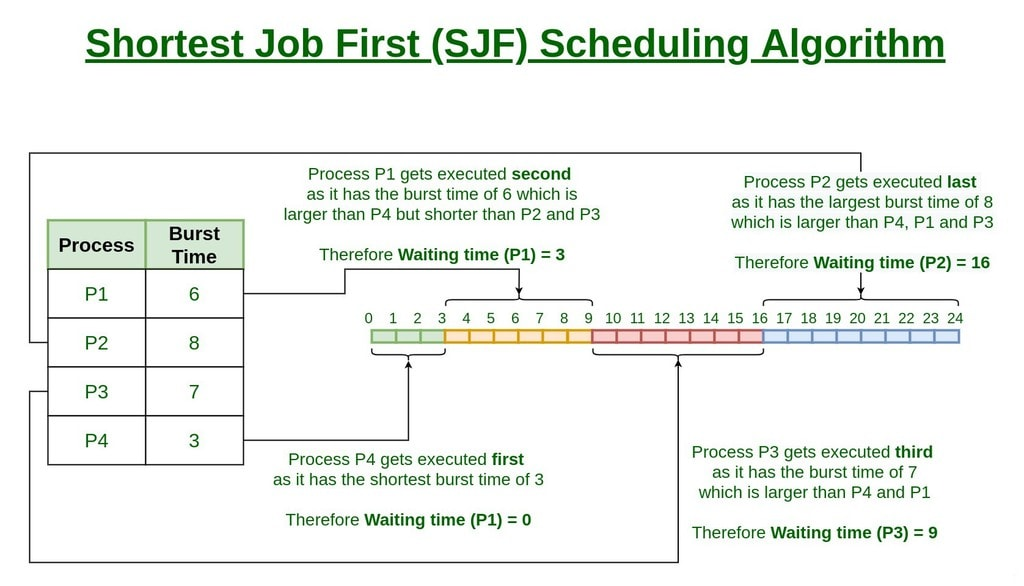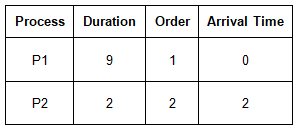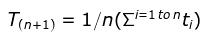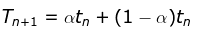Courses

# Shortest Job First (or SJF) CPU Scheduling Notes | EduRev

## GATE : Shortest Job First (or SJF) CPU Scheduling Notes | EduRev

The document Shortest Job First (or SJF) CPU Scheduling Notes | EduRev is a part of the GATE Course Operating System.
All you need of GATE at this link: GATE

Program for Shortest Job First (or SJF) CPU Scheduling

Shortest job first (SJF) or shortest job next, is a scheduling policy that selects the waiting process with the smallest execution time to execute next. SJN is a non-preemptive algorithm.

• Shortest Job first has the advantage of having a minimum average waiting time among all scheduling algorithms.
• It is a Greedy Algorithm.
• It may cause starvation if shorter processes keep coming. This problem can be solved using the concept of ageing.
• It is practically infeasible as Operating System may not know burst time and therefore may not sort them. While it is not possible to predict execution time, several methods can be used to estimate the execution time for a job, such as a weighted average of previous execution times. SJF can be used in specialized environments where accurate estimates of running time are available.

Algorithm

1. Sort all the process according to the arrival time.
2. Then select that process which has minimum arrival time and minimum Burst time.
3. After completion of process make a pool of process which after till the completion of previous process and select that process among the pool which is having minimum Burst time.How to compute below times in SJF using a program?

1. Completion Time: Time at which process completes its execution.
2. Turn Around Time: Time Difference between completion time and arrival time. Turn Around Time = Completion Time – Arrival Time
3. Waiting Time(W.T): Time Difference between turn around time and burst time.
Waiting Time = Turn Around Time – Burst Time

Shortest Remaining Time First (SRTF) scheduling

In the Shortest Remaining Time First (SRTF) scheduling algorithm, the process with the smallest amount of time remaining until completion is selected to execute. Since the currently executing process is the one with the shortest amount of time remaining by definition, and since that time should only reduce as execution progresses, processes will always run until they complete or a new process is added that requires a smaller amount of time.

Shortest Remaining Time First (Preemptive SJF):
Example• P1 waiting time: 4-2 = 2
• P2 waiting time: 0
• The average waiting time(AWT): (0 + 2) / 2 = 1

1. Short processes are handled very quickly.
2. The system also requires very little overhead since it only makes a decision when a process completes or a new process is added.
3. When a new process is added the algorithm only needs to compare the currently executing process with the new process, ignoring all other processes currently waiting to execute.

1. Like shortest job first, it has the potential for process starvation.
2. Long processes may be held off indefinitely if short processes are continually added.

Shortest Job First CPU Scheduling with predicted burst time

Shortest Job First (SJF) is an optimal scheduling algorithm as it gives maximum Throughput and minimum average waiting time(WT) and turn around time (TAT) but it is not practically implementable because Burst-Time of a process can’t be predicted in advance.
We may not know the length of the next CPU burst, but we may be able to predict its value. We expect the next CPU burst will be similar in length to the previous ones. By computing an approximation of the length of the next CPU burst, we can pick the process with the shortest predicted CPU burst.

There are two methods by which we can predict the burst time of the process:

1. Static method: We can predict the Burst-Time by two factors:
• Process size: Let say we have Process Pold having size 200 KB which is already executed and its Burst-time is 20 Units of time, now lets say we have a New Process Pnew having size 201 KB which is yet to be executed.
We take Burst-Time of already executed process Pold which is almost of same size as that of New process as Burst-Time of New Process Pnew.
• Process type: We can predict Burst-Time depending on the Type of Process. Operating System process(like scheduler, dispatcher, segmentation, fragmentation) are faster than User process( Gaming, application softwares ). Burst-Time for any New O.S process can be predicted from any old O.S process of similar type and same for User process.
Note: Static method for burst time prediction is not reliable as it is always not predicted correctly.
2. Dynamic method: Let ti be the actual Burst-Time of ith process and Τn + 1 be the predicted Burst-time for n + 1th process.
• Simple average: Given n processes ( P1, P2… Pn)• Exponential average (Aging)where α = is smoothing factor and 0 <= α <= 1 ,
tn = actual burst time of nth process,
Τn = predicted burst time of nth process.
General term,
αtn + (1 - α)αtn - 1 + (1 - α)2αtn-2...+ (1 - α)jαtn - j...+ (1 - α)n + 1Τ0
Τ0 is a constant or overall system average.

Smoothening factor (α): It controls the relative weight of recent and past history in our prediction.

1. If α = 0, Τn + 1 = Τn i.e. no change in value of initial predicted burst time.
2. If α = 1, Τn + 1 = tn i.e. predicted Burst-Time of new process will always change according to actual Burst-time of nth process.
3. If α = 1 / 2, recent and past history are equally weighted.

Example: Calculate the exponential averaging with T1 = 10, α = 0.5 and the algorithm is SJF with previous runs as 8, 7, 4, 16.
(a) 9
(b) 8
(c) 7.5
(d) None
Ans:
(c)
Explanation: Initially T1 = 10 and α = 0.5 and the run times given are 8, 7, 4, 16 as it is shortest job first,
So the possible order in which these processes would serve will be 4, 7, 8, 16 since SJF is a non-preemptive technique.
So, using formula: T2 = α * t1 + (1 - α)T1
so we have,
T2 = 0.5 * 4 + 0.5 * 10 = 7, here t1 = 4 and T1 = 10
T3 = 0.5 * 7 + 0.5 * 7 = 7, here t2 = 7 and T2 = 7
T4 = 0.5 * 8 + 0.5 * 7 = 7.5, here t3 = 8 and T3 = 7
So the future prediction for 4th process will be T4 = 7.5 which is the option(c).

Offer running on EduRev: Apply code STAYHOME200 to get INR 200 off on our premium plan EduRev Infinity!

69 docs|23 tests

,

,

,

,

,

,

,

,

,

,

,

,

,

,

,

,

,

,

,

,

,

;